# MP Board Class 7th Maths Solutions Chapter 6 The Triangles and Its Properties Ex 6.3

## MP Board Class 7th Maths Solutions Chapter 6 The Triangles and Its Properties Ex 6.3

Question 1.
Find the value of the unknown x in the following diagrams: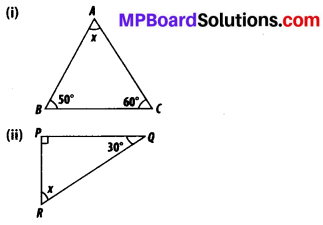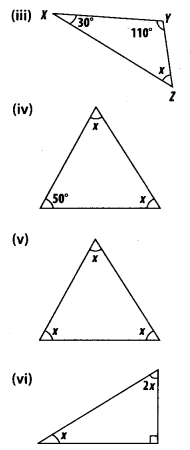Solution:
The sum of all interior angles of a triangle is 180°. By using this property, these problems can be solved as follows :
(i) x + 50° + 60° = 180°
⇒ x +110° = 180°
⇒ x = 180° -110° = 70°

(ii) x + 90° + 30° = 180°
⇒ x + 120° = 180°
⇒ x = 180° – 120° = 60°

(iii) x + 30° + 110° = 180°
⇒ x + 140° = 180°
⇒ x = 180° – 140° = 40°

(iv) 50° + x + x = 180°
⇒ 2x = 180° – 50° = 130°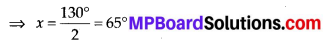(v) x + x + x = 180°
⇒ 3x = 180°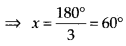(vi) x + 2x + 90° = 180°
⇒ 3x = 180° – 90° = 90° = 90°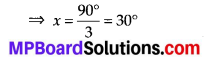Question 2.
Find the values of the unknowns x and y in the following diagrams: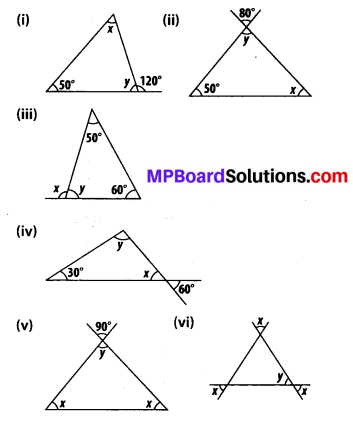Solution:
(i) y + 120° = 180° (Linear pair)
⇒ y = 180° – 120° = 60°
Now, x + y + 50° = 180°
(Angle sum property)
⇒ x + 60° + 50° = 180° x = 180° – 60° – 50° = 70°

(ii) y = 80° (Vertically opposite angles)
Now, y + x + 50° = 180°
(Angle sum property)
⇒ 80° + x + 50° = 180°
⇒ x = 180° – 80°- 50° = 50°

(iii) y + 50° + 60° = 180°
(Angle sum property)
⇒ y = 180° – 60° – 50° = 70°
Now, x + y = 180° (Linear pair)
⇒ x = 180° – y = 180° – 70° = 110°

(iv) x = 60° (Vertically opposite angles)
Now, 30° + x + y = 180°
(Angle sum property)
⇒ 30° + 60° + y = 180° y = 180° – 30° – 60° = 90°

(v) y = 90° (Vertically opposite angles)
Now, x + x + y = 180°
(Angle sum property)
⇒ 2x + 90° = 180°
⇒ 2x = 180° – 90° = 90°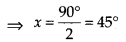(vi) y = x (Vertically opposite angles)
a = x (Vertically opposite angles)
b = x (Vertically opposite angles)
Now, a + b + y = 180°
(Angle sum property)
⇒ x + x + x= 180°
⇒ 3x = 180°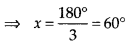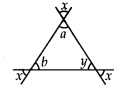Hence, y = x = 60°